Electrochemical (2017 VCE) 1) Lithium-ion rechargeable batteries are used in mobile phones. Environmental conditions can affect the number of charge cycles for a lithium-ion battery until the end of its useful life. Which of the following environmental conditions would be expected to result in the largest number of charge cycles for a lithium-ion battery?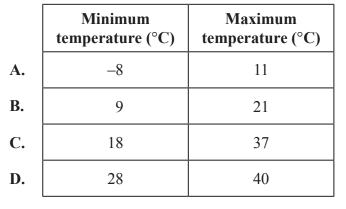Solution2) A galvanic cell consists of two connected half-cells that can produce an electron flow. Which combination of standard half-cell pairs would be expected to result in a cell potential of 1.41 V?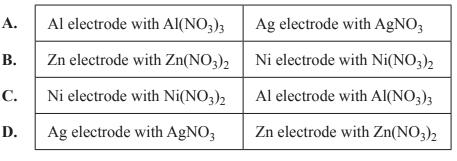Solution3) The reaction below represents the discharge cycle of a standard lead-acid rechargeable car battery.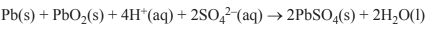During the recharge cycle, the pH A. increases and solid Pb is a reactant. B. increases and solid PbO2 is produced. C. decreases and chemical energy is converted to electrical energy. D. decreases and electrical energy is converted to chemical energy. Solution4) An increasingly popular battery for storing energy from solar panels is the vanadium redox battery. The battery takes advantage of the four oxidation states of vanadium that are stable in aqueous acidic solutions in the absence of oxygen. A schematic diagram of a vanadium redox battery is shown below.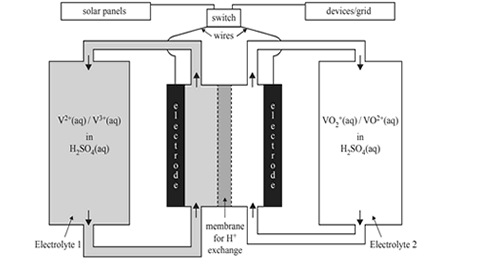The two relevant half equations are given below.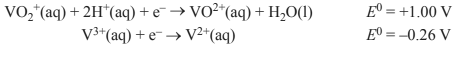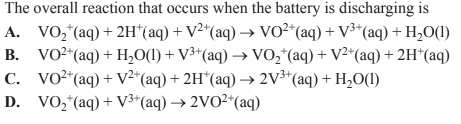Solution   If air is present, the following half-equations are also relevant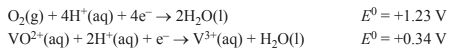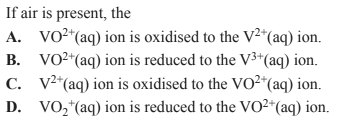Solution